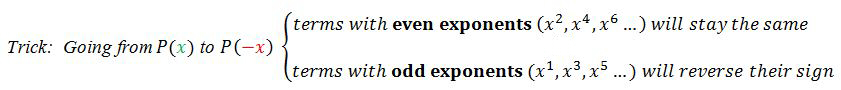# Descartes' rule of signs#### Everything You Need in One Place

Homework problems? Exam preparation? Trying to grasp a concept or just brushing up the basics? Our extensive help & practice library have got you covered.#### Learn and Practice With Ease

Our proven video lessons ease you through problems quickly, and you get tonnes of friendly practice on questions that trip students up on tests and finals.#### Instant and Unlimited Help

Our personalized learning platform enables you to instantly find the exact walkthrough to your specific type of question. Activate unlimited help now!##### Intros
###### Lessons
1. Introduction to Descartes' Rule of Signs
2. Fundamental Theorem of Algebra VS. Descartes' Rule of Signs
3. Descartes' Rule of Signs – explained.
exercise: Use Descartes' Rule of Signs to determine the possible combinations of roots of:
$P(x)=2x^6-7x^5+x^4+5x^3-6x^2-10$
##### Examples
###### Lessons
1. Use Descartes' Rule of Signs to Determine the Number of Positive and Negative Roots
Use Descartes' Rule of Signs to determine the possible number of positive roots and negative roots:
1. $P(x)=4x+9x^6-5x^2-10x^7+6x^3-8x^5-7x^4+3$
2. $P(x)=x^4-5x^2-6x$ (note: NO constant term!!)
2. Use the Rational Roots Theorem, together with Descartes' Rule of Signs, to Find Roots Effectively
Solve:
1. $-3x^3+22x^2-37x+10=0$
2. $-3x^3-5x-7x^2+2x^4-3=0$
###### Topic Notes
Descartes' Rule of Signs For a polynomial $P(x)$:
$\bullet$ the number of positive roots = the number of sign changes in $P(x)$, or less than the sign changes by a multiple of 2.
$\bullet$ the number of negative roots = the number of sign changes in $P(-x)$, or less than the sign changes by a multiple of 2.

Note: Before applying the Descartes' Rule of Signs, make sure to arrange the terms of the polynomial in descending order of exponents.